# Deep Latent Factor Models and Variational Inference with Backprop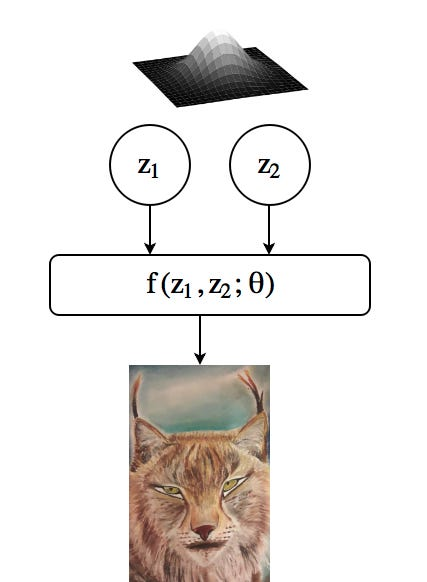Multivariate normally distributed factors generate the image together with complex f(.) function. (Just an illustration of a process)

Materials around us, processes and occasions we are witnessing, datasets we have are all our observations and there are generative models in nature that we can not observe but generate these observations. Latent Factor Models describe our belief on generative process of observations, probabilisticly. Today’s topic is deep latent factor models which are the statistical models that try to explain the high dimensional complex datas with low dimensional (hopefully interpretable) factors. In deep latent factor models we assume that there is a complex function which accepts few underlying factors as input and generates the complex outputs which we observe.

One may has various motivations to use latent factor models. Interpretability is clearly one of them. Imagine that we find the interpretable factors that generate the face images. We have the factors that specify the shape of hair, factors that generates the color of eye, and factors that creates the gender. If we had these factors, we would be able to generate completely new faces by specifying some of their attributes. You may say that GANs also generate new unseen faces. But remember GANs take samples from the estimated probability distribution, completely randomly, there is no attribute specification in GANs (at least as far as I know. there are tons of GAN models out there and it is really hard to be up to date with all the recent developments in AI). Breaking the curse of dimensionality is another motivation. If the dimensionality of datas is large and we want to train a classification model, number of parameters which needs to be learned is also high and requires lots of data for a well generalized model. By reducing dimensionality, we also decreases the number of parameters so fewer data is required for learning. Dimensionality reduction is also useful for visualization, especially if the dimension can be reduced to 2 or 3. These are just the few of motivations to use latent factor models.

Quick Note: Some knowledge of Expectation Maximization algorithm is prerequisite to understand the rest of the article. A wonderful chapter with clear insights and elegant math can be found in Bishop’s Pattern Recognition and Machine Learning book.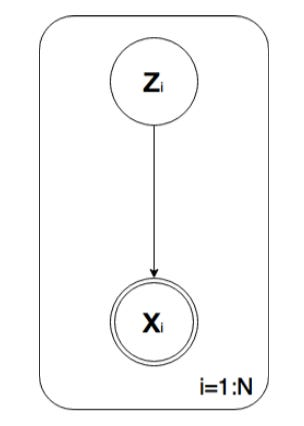A general graphical representation of latent factor models. z represents latent variable vector. x is observation vector and there are N observations.

In the beginning, we assume that there are model parameters theta and we want to apply maximum likelihood parameter estimation which is setting the parameters such that the likelihood distribution reaches to the maximum. If we knew the z values for each datas, it would be rather easy task to estimate parameters(for more info, please take a look at my GLM article). Remember, one of our tasks is to estimate parameters which maps z values to x values. If we do not know z values, how can that be possible? Well, EM algorithm offers us a way to achieve it by applying Expectation and Maximization steps iteratively. Let’s dive into math by defining the objective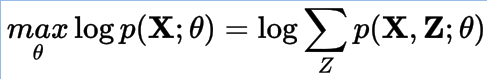Maximum likelihood objective function where X and Z represent all the observations and latent variables respectively. Theta is model parameter(s).

Unfortunately, the aboveobjective contains an intractable sum operation due to exponentially growing elements that needs to be summed. In order to overcome this problem, we introduce a q(Z) term and utilize Jensen’s inequality to find a lower bound to loglikelihood.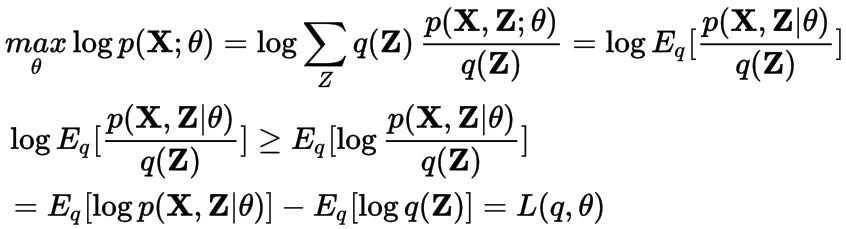Lower bound to log-likelihood which is a function of q and theta

Motivation behind the finding lower bound to loglikelihood is that although we can’t evaluate loglikelihood directly, we may evaluate lower bound so that we may gain information about loglikelihood. For this purpose, we rearrange the lower bound term and write it as summation of loglikelihood term and an additional divergence term.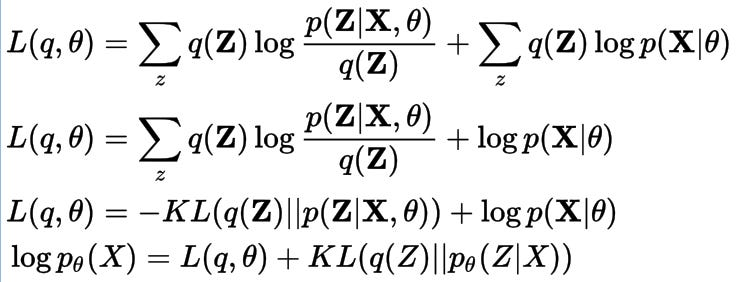loglikelihood can be written as summation of Lower bound and reverse-KL divergence terms

KL divergence is an asymmetric metric that measures the divergence between two probability distributions and takes the value of 0 as the minimum value when two distributions are the same. As you can see, when the KL divergence takes its minimum value, loglikelihood becomes equal to lower bound. Therefore we need to equalize q(Z) to posterior of Z p(Z|X) to make KL divergence 0. Let’s rewrite lower bound by using p(Z|X) instead of q(Z).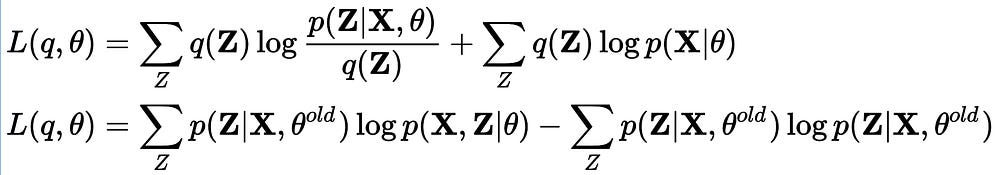Changing the q(Z) terms with the posterior of Z which is calculated for the old theta parameters

Right now, we can evaluate the value of loglikelihood for the old theta parameters and it is basically equal to lower bound value for old theta. But the lower bound value can be increased by optimizing the theta values. The second term of lower bound only includes old theta parameters and there is nothing to do for that part. But the first part can be further optimized and value of lower bound can be increased. This also means that log-likelihood can be increased further by estimating new theta values. When you look at the first part carefully, you can see that it is an expectation term and evaluating this expectation term forms the E-part of EM algorithm. E-step includes the inference of Z’s posterior, so we can rename E-step with inference step. Maximizing the lower bound by estimating new model parameters is called M-step, but I think there is nothing wrong with calling it learning step. I preferred to rename E and M steps by considering the upcoming parts of the article.

It is not always easy to find the posterior of latent factors, especially when we are dealing with complex models. This time, instead of computing the posterior exactly, we will approximate to it by a proposal distribution q(z) with variational parameters phi. For this case, we assume that there are no model parameters.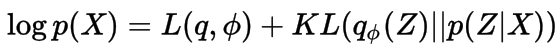We assume there are no model parameters theta, and we approximate to p(Z|X) by tuning variational parameters phi.

In this case, loglikelihood is constant and does not vary with q and phi choices, but we can not evaluate it directly, again. What we need to do is to choose a variational distribution q smartly(we try to choose a distribution family as much as similar to the posterior of z) and optimize its parameters phi in order to decrease the KL divergence between original posterior and proposal distribution. In other words, we approximate to loglikelihood with a lower bound by tuning its inputs q and phi. Finding the function q is the topic of variational calculus but for fully factorized q(mean field approximation), the form of q is already known(for more info take a look at Bishop’s book). This process is called Variational Bayes.

In the next part, we will dive into the details of variational inference for deep latent factor models and I will introduce famous Variational Autoencoder. Before working on that we will rearrange the lower bound equations a little bit.

What if the model also has parameters? Then the lower bound turns into the function of q, theta, and phi.

In order to make the problem simpler, we can design the model carefully by considering conjugacy and use fully factorized q function to not to optimize the objective function with respect to q. So the lower bound needs to be optimized w.r.t model parameters theta and variational parameters phi. Estimation of the model parameters is nothing but the M-step of EM algorithm. On the other hand, variational parameter estimation is related to finding the posterior of latent variables, so it is an inference step and forms the E-step of EM algorithm. We call this overall process Variational EM.

Now consider a complex model with Gaussian likelihood where the mean and the covarince are neural networks that accept the latent factors z as inputs. We also define a prior distribution on latent factors as the following.

It is really hard to make an efficient inference in such a complex model with classical variational inference methods. The recent advances in variational inference enable us to make inference in these complex models. In the above model, the model parameters(theta) that we would like to estimate are nothing but the weights of the neural networks.

For the inference, we specify the Gaussian distribution as variational distribution family but this time we define neural network structures which maps the observation to the mean and covariance of the variational distribution(we may prefer to not to use observations and inference networks but it is wise to use them while we can). So the objective in the Variational EM part tuns into

It is quite interesting to observe that every latent feature(z_i) has its own variational parameters(mean and covariance matrix) as it should be, but these parameters are generated by observations(x_i) and global variational parameters(phi) which are weights of the inference networks.

Deep learning society prefers to use loss functions as objectives, so instead of maximizing lower bound we can minimize its negative.

The loss function defines an autoencoder because its first term tries to reconstruct the observations which refers to decoder and the second term encodes observations to latent representations by trying to keep it close to the prior as much as possible.

The second term of the loss function is the KL divergence between two multivariate Gaussians and we know its form. The expression depends on variational parameters phi and the term will be optimized w.r.t. phi with stochastic gradient descent.

The first term is an expectation and instead of calculating it analytically(we can not), we can estimate it with Monte Carlo integration. The problem here is that after sampling z from q(z|x), we lose all the connection with variational parameters and cannot optimize the term w.r.t. variational parameters although the term depends on them. Reparametrization trick is cool method to overcome the problem.

In reparametrization trick, we write the sample z as the output of a deterministic function with parameters mu and Sigma, and input epsilon which is a sample from zero mean unit variance Normal distribution. Now we can optimize the term w.r.t. variational parameters with chain rule(dE/dz * dz/dphi).

In this article, I tried to explain the very basic idea of Variational Autoencoder starting from the well known EM algorithm. It was quite interesting to see that by introducing an inference network with a small update in the objective function, we reached a special type of regularized autoencoder and this enabled us to run the E and M steps of Variational EM with backpropagation algorithm.

### References

Kingma, Diederik P., and Max Welling. “Auto-encoding variational bayes.” arXiv preprint arXiv:1312.6114 (2013).

Bishop’s Pattern Recognition and Machine Learning book

EM and Variational Inference

Source: Deep Learning on Medium